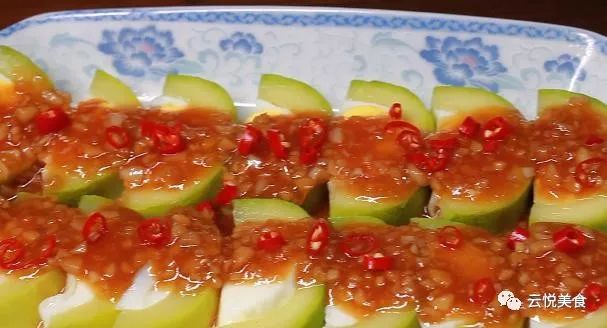# 西葫芦里面加4个鸡蛋，不用炒不凉拌，招待客人，比吃肉都过瘾

2019-10-22' data-lazy='1' data-height='332' data-width='618' width='618' height='auto'>' data-lazy='1' data-height='365' data-width='613' width='613' height='auto'>' data-lazy='1' data-height='368' data-width='620' width='620' height='auto'>' data-lazy='1' data-height='330' data-width='614' width='614' height='auto'>' data-lazy='1' data-height='327' data-width='617' width='617' height='auto'>' data-lazy='1' data-height='342' data-width='608' width='608' height='auto'>' data-lazy='1' data-height='326' data-width='622' width='622' height='auto'>' data-lazy='1' data-height='320' data-width='615' width='615' height='auto'>' data-lazy='1' data-height='328' data-width='607' width='607' height='auto'>